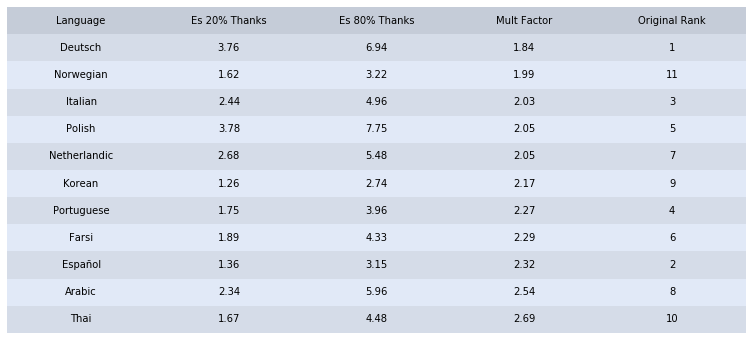#### Introduction¶

The goal of this analysis was to see how many editors are involved in sending thanks. We do this by setting inspection points at 20% and 80% and calculating the percentage of editors responsible for these two percentages of thanks.

#### ICDF Part 1¶

• Count the number of editors in a timeframe use PROJECT;

select count(distinct rev_user) as num_editors from revision where (rev_timestamp < timestamp('2018-06-01') and rev_timestamp >= timestamp('2017-06-01') and rev_user != 0);

#### ICDF Part 2¶

• Count the number of editors responsible for some percentage of thanks use PROJECT;

select count(distinct log_user) as num_thankers from logging_userindex where (log_action = 'thank' and log_type='thanks' and log_timestamp < timestamp('2018-06-01') and log_timestamp >= timestamp('2017-06-01') and rand() > 1-x);

Note: returns data for x*100 percent of thanks, eg to get 20% of thanks, set rand() > 0.8

import csv

total_editors = []
editors_by_thanks = []
languages = []
#define filename
input_file = '(1-2)-data/editors-by-thanks-sample.csv'


Note: The SQL queries will return csvs with a single number. To use this pipeline, you will have to manually amalgamate the data.

#get data from csv (which was manually created)
def get_data(languages=languages, editors_by_thanks=editors_by_thanks, total_editors=total_editors, input_file=input_file):
with open(input_file, 'r', encoding='utf-8') as csvfile:
rder = csv.DictReader(csvfile)
for row in rder:
languages.append(row['Language'])
editors_by_thanks.append([int(row['80 Percent Thanks']), int(row['20 Percent Thanks'])])
total_editors.append(int(row['Editors']))

get_data()

def to_editor_percentages(denominator, numerators):
#convert absolute numbers of editors to percentages
lst = []
for x in numerators:
lst.append(x*100/denominator)
return lst

#order the columns and round all floats (so the graph looks nicer)
def format_data(editors=total_editors, editors_by_thnx=editors_by_thanks, languages=languages):
splits = []
for i in range(0, len(editors)):
lst = to_editor_percentages(editors[i], editors_by_thnx[i])
lst = [lst, lst]
lst.append(lst/lst)
lst.append(i+1)
lst = [round(x, 2) for x in lst]
splits.append([languages[i]] + lst)
return splits

data = format_data(total_editors, editors_by_thanks, languages=languages)
#sort data by fourth column
data = sorted(data, key=lambda tupl: tupl)

import numpy as np
import pandas as pd
import matplotlib.pyplot as plt

fig, ax = plt.subplots()

#hide axes
ax.axis('off')
ax.axis('tight')

#styling -- color cells by row
colors = [['#e1e9f7']*len(data)]*len(data)
for i in range(0, len(colors)):
if (i % 2) == 0:
colors[i] = ['#d5dce8']*len(data)

df = pd.DataFrame(data, columns=['Language', 'Es 20% Thanks', 'Es 80% Thanks', 'Mult Factor', 'Original Rank'])

table = ax.table(cellText=df.values, cellColours=colors, colColours=['#c5ccd8']*len(df.columns), colLabels=df.columns, loc='center', cellLoc='center')

#styling -- get rid of lines in table
d = table.get_celld()
for k in d:
d[k].set_linewidth(0)

table.scale(2, 2)

fig.tight_layout()

plt.savefig('../figures/icdf-thanker-population.png', bbox_inches='tight')
plt.show()#### Conclusion¶

Around twice as many editors are responsible for four times as many thanks, indicating that the rate of increase in editor percentage decreases as thanks percentage increases.